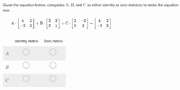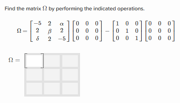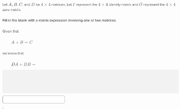The Zero and identity matrices exercise appears under the Precalculus Math Mission and Mathematics III Math Mission. This exercise explores the properties of the zero and identity matrices.

## Types of Problems

There are four types of problems in this exercise:

1. Which matrices are the identity matrix or the zero matrix: This problem has a matrix equation with some variables. The user is asked to determine which of the variables are the identity and which are the zero matrix.Which matrices are the identity or the zero matrix
2. Find the result of the matrix expression: This problem provides a matrix expression which involves zero and identity matrices. The user is expected to find the result of the expression and provide it in the matrix template.Find the result of the matrix expression
3. Simplify the expression: This problem provides some information about matrices and an algebraic expression. The user is expected to find the result of the algebraic expression and write it in terms of the variables.Simplify the expression
4. Find the result of each product: This problem provides a matrix and a select an option grid. The user is expected to find the result of each expression and select the option that is the correct evaluation of each expression.Find the result of each product

## Strategies

Knowledge of the mechanics behind calculating determinants is encouraged to ensure success on this exercise.

1. The zero matrix consists of all zero entries. When it is multiplied against a matrix, the result is a matrix with all zero entries.
2. The identity matrix only makes sense with square matrices. It has ones along the diagonal and zeroes elsewhere. When multiplied against a matrix, the result is that the original matrix is returned.
3. A matrix times itself is the zero matrix, the identity matrix, or is idempotent. Most matrices are not idempotent sois not usually.

## Real-life Applications

1. Matrices are the foundational concept in linear algebra.
2. Matrices can be a method for efficiently solving systems of linear equations.
3. Matrices can be viewed as an extension of a number system.